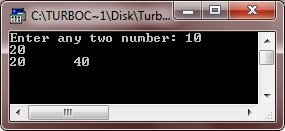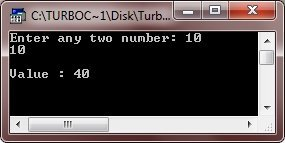# C++ Scope Rules

The program part(s) in which a particular piece of code or a data value (e.g., variable) can be accessed is known as the piece-of-code's or variable's scope.

The scope rules of a language are the rules that decide, in which part(s) of the program a particular piece of code or data item would be known and can be accessed therein.

There are four kinds of scopes in C++ :

• local scope
• function scope
• file scope
• class scope

## C++ Local Scope

A name declared in a book (i.e., {....}) is local to that block and can be used only in it and the other blocks contained under it. The names of formal arguments are treated as if they were declared in the outermost block of that junction.

## C++ Function Scope

The variables declared in the outermost block of a function have function scope i.e., they can be accessed only in the function that declares them. Also labels (of goto) have function scope i.e., they cannot be used outside the function.

## C++ File Scope

A name declared outside all blocks and functions has file scope i.e., it can be used in all the blocks and functions written inside the file in which the name declaration appears.

## C++ Class Scope

A name of a class member has class scope and is local to its class.

### C++ Scope Rules Example

Let's Look at the following example :

```#include<......>
int x, y;          // file scope (Global Variables)
void main()
{
clrscr();
int a, b;       // function scope for main()
float amt;		// local variables of main()

void check(int);
:
{
char grade;    // block scope (local variable of block)
:              // block 2
}
}
void check(int i)      // function scope for check()
{
long temp;         // local variables of check()
}```

Here is an example program, demonstrating scope rules in C++

```/* C++ Scope Rules */

#include<iostream.h>
#include<conio.h>

int a, b;    // global variables
int fun(int);

void main()
{
clrscr();
int num1, num2;    // local variables of main()
cout<<"Enter any two number: ";
cin>>num1>>num2;
if(num1==num2)
{
int temp;   // block scope (local variable of this if block)
temp = num1 + num2;
cout<<"\nValue : "<<fun(temp);
}
else
{
cout<<fun(num1)<<"\t"<<fun(num2);
}
getch();
}
int fun(int x) 		// function scope for fun()
{
int res;	// local variable of fun()
res = x * 2;
return res;
}```

Here are the two sample runs of the above C++ program:Tools
Calculator

Quick Links
Signup - Login - Give Online Test